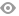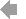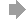﻿ Page 6 - MATINF Nr. 6
Basic HTML VersionView Full Version
Page 6 - MATINF Nr. 6
P. 6

``````6                                                                                E.A. Jos´e Garc´ıa

Fig. 1: A cyclic quadrilateral ABCD.

Proof. First we will ﬁnd an expression for cos α in terms of a, b, c and d. Let ∠BCD = γ. By
the Law of Cosines and keeping in mind that α and γ are supplementary, we have

◦
2
2
2
2
a + d − 2ad cos α = b + c − 2bc cos (180 − α).
2
2
2
Yielding cos α =  a +d −b −c 2  . Now, making use of the half angle formula for cosine,
2
2
2
α     2ad + 2bc + a + d − b − c   2
cos 2  =                                                                   (2)
2
(a + d) − (b − c) 2
=
(a + b − c + d)(a − b + c + d)
=                                                                   (3)
1     a + b + c + d       a + b + c + d   
=                         − c                  − b
(s − b)(s − c)
=                .

The other formulae can be obtained similarly by replacing cos  2 α  by 1 − sin 2 α  in (2).
2             2
In personal email communication with Peter Doyle , the renowned mathematician J. H.
Conway has given the same proof of Heron’s formula that we present here. However, as the aim
of this paper is to present these area theorems as mere links of a chain of related theorems from
a new standpoint, we deduce (4) in a diﬀerent way by just setting c = 0 in (1).
Here, ∆ 0 , ∆ 1 , ∆ 2 and ∆ 3 stand for the areas of a triangle, a cyclic quadrilateral, a bicentric1   2   3   4   5   6   7   8   9   10   11